﻿ 基于层次分析法的房地产价格模型研究及应用

# 基于层次分析法的房地产价格模型研究及应用The Study and Applications of Property Price Model Based on Hierarchical Analysis

Abstract: China’s real estate industry has entered a new era of sustained and rapid development. As a result, the price of real estate went up. At the same time, real estate bubble emerged in cities which made urban housing prices become a hot issue among the public. The author analyzed the factors of this issue and made suggestions as follows: firstly, a large amount of real estate price data was collected. According to analyzing difference among the data, nine factors that could affect real estate prices were found out. Then, the author used AHP (Analytic Hierarchy Process) to establish a model of level analysis. This model determined three main factors that influenced property price. Then the paper confirmed the model’s validity through applicability and consistency testing. Secondly, according to the collected data and the conclusion which made by AHP, a multiple linear regression model was established. The least square method was used to solve the model and obtain model parameters. Then, the predictions of the model were tested. Besides, the extreme testing proved its reliability. Finally, targeting at the present situation of real estate market in China and conclusion of the model, suggestions are given in this paper. These suggestions can be used in many ways: policies on land resources, market and other policies. These suggestions also gave the government ways to control real estate bubble.

1. 引言

2. 房地产价格层次分析模型的建立

2.1. 模型假设

1) 不考虑外在自然因素对房价成本的影响；

2) 本文所用数据都来自于各城市普通居民住宅区，不包括别墅区域和高级住宅区域；

3) 不考虑投资者或居民因个人因素造成的房地产价格差异；

4) 所得数据波动都在合理的范围内并且最近不会有太大的经济变化影响到房地产价格；

5) 不考虑资源分布不均以及资源枯竭等问题；

6) 全国各城市影响房地产价格的因素基本上是一致的。

2.2. 符号说明

P：全国房屋平均价格；

x1：土地建设的成本(土地本身的资源价值和建设材料的成本)；

x2：全国居民人均可支配收入；

x3：国民生产总值GDP;

${\omega }_{i}$ ：权向量；

$\lambda$ ：对应矩阵最大特征根。

2.3. 构建层次分析模型

(1) 顶层(目标层C)为房价；

(2) 中间层(准则层O)：O1：房地产商期望；O2：消费者需求；O3：国家政策；

(3) 低层(方案层M)层：M1：土地及建设的成本；M2：全国GDP总量；M3：投机行为；M4：居民收入；M5：人口密度；M6：住房环境；M7：货币政策；M8：土地政策；M9：税收政策。

1) 对目标层C和准则层O建立权重判断矩阵 $C=\left\{{O}_{1},{O}_{2},{O}_{3}\right\}$ ，它表示准则中3个影响因素(房地产商的期望、消费者的需求和国家的相关政策)。

$A=\left[\begin{array}{ccc}1& 2& 4\\ \frac{1}{2}& 1& 2\\ \frac{1}{4}& \frac{1}{2}& 1\end{array}\right]$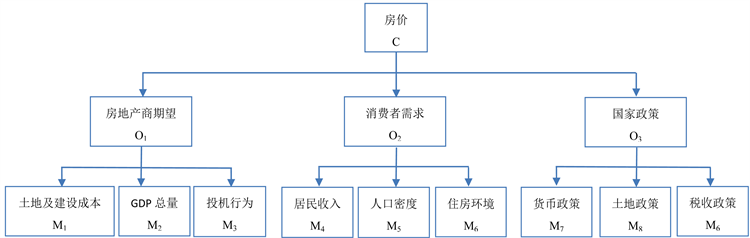Figure 1. Model of hierarchical analysis model

2) 对准侧层O1(房地产商期望)和方案层M建立权重判断矩阵 ${O}_{1}=\left\{{M}_{1},{M}_{2},{M}_{3}\right\}$ ，它表示准则中3个影响因素(建设成本、全国GDP总量和投机行为)。

$B=\left[\begin{array}{ccc}1& 2& 3\\ \frac{1}{2}& 1& 2\\ \frac{1}{3}& \frac{1}{2}& 1\end{array}\right]$

${\omega }_{2}={\left(0.5396,0.2970,0.1634\right)}^{Τ}$ ，其最大特征值为 $\lambda =3.0092$$CR=\frac{CI}{RI}=0.00\text{79}<0.\text{1}$ ，组合一致性检验通

3) 对准侧层O2(消费者需求)和方案层M建立权重判断矩阵 ${O}_{2}=\left\{{M}_{4},{M}_{5},{M}_{6}\right\}$ ，它表示准则中3个影响因素(居民收入、人口密度和住房环境)。

$C=\left[\begin{array}{ccc}1& 3& 5\\ \frac{1}{3}& 1& 3\\ \frac{1}{5}& \frac{1}{3}& 1\end{array}\right]$

${\omega }_{3}={\left(0.6578,0.2320,0.1102\right)}^{Τ}$ ，其最大特征值 $\lambda =2.8955$$CR=\frac{CI}{RI}=0.0901<0.1$ ，组合一致性检验通过。

4) 对准侧层O3(国家政策)和方案层M建立权重判断矩阵 ${O}_{3}=\left\{{M}_{7},{M}_{8},{M}_{9}\right\}$ ，它表示准则中3个影响因素(货币政策、土地政策和税收政策)。

$D=\left[\begin{array}{ccc}1& 4& 6\\ \frac{1}{4}& 1& 4\\ \frac{1}{6}& \frac{1}{4}& 1\end{array}\right]$

${\omega }_{4}={\left(0.6817,0.2363,0.0819\right)}^{Τ}$ ，其最大特征值 ${\lambda }_{}=3.1079$$CR=\frac{CI}{RI}=0.0930<0.1$ ，组合一致性检验通过。

3. 多元回归模型的建立与求解

3.1. 土地建设成本与房价的一元线性回归模型

$y=\text{51}.\text{174}0+\text{1}.\text{312}0x$

3.2. 人均可支配收入与房价的一元线性回归模型

$y=760.9610+0.2129x$Table 2. Cost of land construction and national average price of home from 2002 to 2012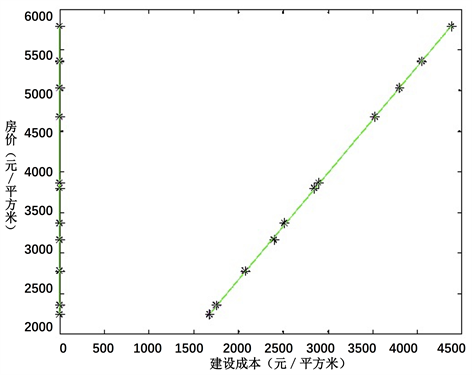Figure 2. Cost of land construction and national average price of homeTable 3. Per-capita disposable income and national average price of home from 2002 to 2012  

3.3. 全国GDP总量与房价的一元线性回归模型

$y=1329.3+0.00809x$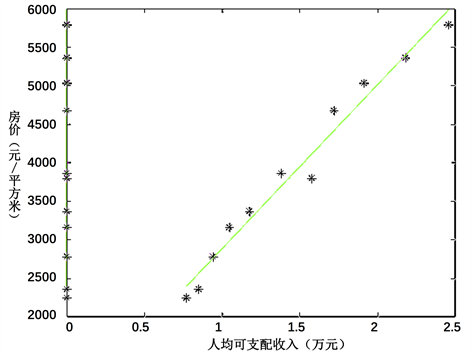Figure 3. Per-capita disposable income and national average price of homeTable 4. GDP and national average price of home from 2002 to 2012  

3.4. 房价的多元线性回归模型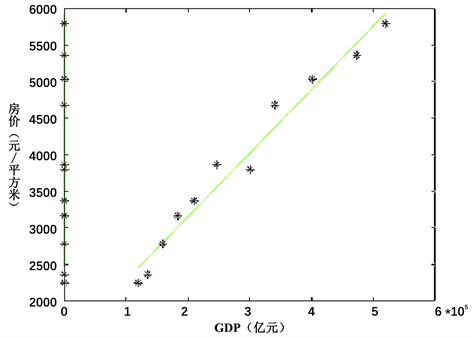Figure 4. GDP and national average price of home

$P={b}_{0}+{b}_{1}{x}_{1}+{b}_{2}{x}_{2}+{b}_{3}{x}_{3}+e$

$P=23.7983+1.2923{x}_{1}+0.0154{x}_{2}+0.0003{x}_{3}$

3.5. 房价的多元线性回归模型检验

$P=23.7983+1.2923{x}_{1}+0.0154{x}_{2}+0.0003{x}_{3}$

4. 抑制房地产泡沫合理化建议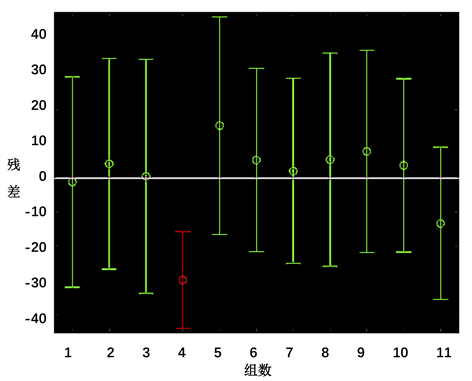Figure 5. Residual plot of multiple linear regressions

1) 完善我国住房保障制度，积极发展住房租赁市场。我国经济飞速发展的同时也带动了房价的飞速发展，同时，我国也是一个人口大国。因此，完善住房保障制度，积极发展住房租赁市场就显得尤为重要  。加大商品房市场、二手房市场、廉租房市场等的新建，那么，将缓解我国因人口众多导致住房供不应求而引发的房价居高不下的现象。

2) 强化土地资源管理，严控土地价格。政府应该强化对土地资源管理，调整土地资源的供应量，避免土地供应的过多或过少  。严格控制高档住宅的盲目开发和大规模建设，防止出现过剩的现象，将土地开发权分配到最合理的地方。

3) 调控居民可支配收入。居民可支配的收入是影响我国居民生活水平的关键因素之一。政府可以通过调控居民的可支配收入从而改变居民的消费理念，引导居民理性消费。例如，可以在一些行业给予政策上的支持，引导居民把房地产投资逐渐地转移到其他领域和产业。

4) 严格金融监管力度。调整房地产业相关的银行贷款政策，严格监管审查力度是非常必要的。收缩银行对房地产业的贷款规模，可以促使部分投资者因资金不足而放弃对房地产业的投资，从而降低房地产业的畸形发展，抑制房产泡沫的过度膨胀  。

5) 建立全国统一的房地产市场运行预警预报制度。房地产市场的变化性和不定性常常会给房地产市场的宏观调控带来非常大的困难。因此，及时地收集、整理出房地产市场的相关信息就显得尤为重要  。政府可以通过数据进行一系列合理地分析，并根据当前市场的运行情况来进行房地产市场运行情况的评价和预测。同时，根据所得的最新分析报告和市场的变化情况及时调整相关的政策，将房地产市场泡沫抑制在一定范围内，防止其过度膨胀。

6) 引导民众树立正确的房地产价值观。房地产市场一直居高不下和民众自身的观念有很大的关系。改革开放以来，我国人口急剧增加。人口的增多必然导致用房数量的增加，于是房价也随人口增长而不断增长。例如“有车有房”、“一人多房”的概念在一些地区成了象征财富和经济实力的一种标准，这一定程度上导致了房地产资源的浪费，加剧了房地产泡沫  。因此，引导民众树立正确的房地产价值观就显得尤为重要，在供需的市场关系中，引导民众树立正确的房地产价值观可以从根源上解决房地产价格过高的问题，抑制房地产泡沫。

5. 结语

 刘民权, 孙波. 商业地价形成机制、房地产泡沫及其治理[J]. 金融研究, 2009(10): 22-37.

 吴冠岑, 王沁颖. 我国大中城市房地产泡沫测度与分化研究——以中国35个大中城市为例[J]. 价格理论与实践, 2017(5): 53-56.

 单钰淇, 董佩鑫, 刘超. 层次分析法在制动器惯性台架电模拟效果评估中的应用[J]. 煤炭技术, 2010, 29(6): 187-188.

 国土资源网[EB/OL]. http://www.mlr.gov.cn/

 中华人民共和国国家统计局[EB/OL]. http://data.stats.gov.cn/

 Li, X., Sha, J. and Wang, Z.L. (2017) A Comparative Study of Multiple Linear Regression, Artificial Neural Network and Support Vector Machine for the Prediction of Dissolved Oxygen. Hydrology Research, 48, 1214-1225.
https://doi.org/10.2166/nh.2016.149

 Grégoire, G. (2014) Multiple Linear Regression. European Astronomical Society Publications Series, 66, 45-72.

 Slinker, B.K. and Glantz, S.A. (2008) Multiple Linear Regression. Circulation, 117, 1732-1737.
https://doi.org/10.1161/CIRCULATIONAHA.106.654376

 王雪峰. 房地产泡沫和金融不安全——日本泡沫经济15周年评述[J]. 现代日本经济, 2007, 153(3): 25-29.

 Levitin, A.J. and Wachter, S.M. (2013) The Commercial Real Estate Bubble.

 彭山桂, 程道平, 张勇. 地方政府土地出让策略互动行为的检验及其影响分析[J]. 中国人口•资源与环境, 2017, 27(7): 111-119.

 姬智. 浅谈中国当前的房地产泡沫[J]. 时代金融, 2017(6): 217.

 顾汉龙, 冯淑怡, 王秋兵. 市场机制引入对城镇新增建设用地配置效率的影响[J]. 中国人口•资源与环境, 2017, 27(7): 101-110.

 Chovanec, P. (2011) China’s Real Estate Bubble May Have Just Popped. Foreign Affairs.

 Yan-Ming, Z.A.P.F. (2011) Measuring Real Estate Price Bubble Based on Kalman Filter: An Evidence from Beijing Market. Finance and Trade Research, 22, 59-65.

Top